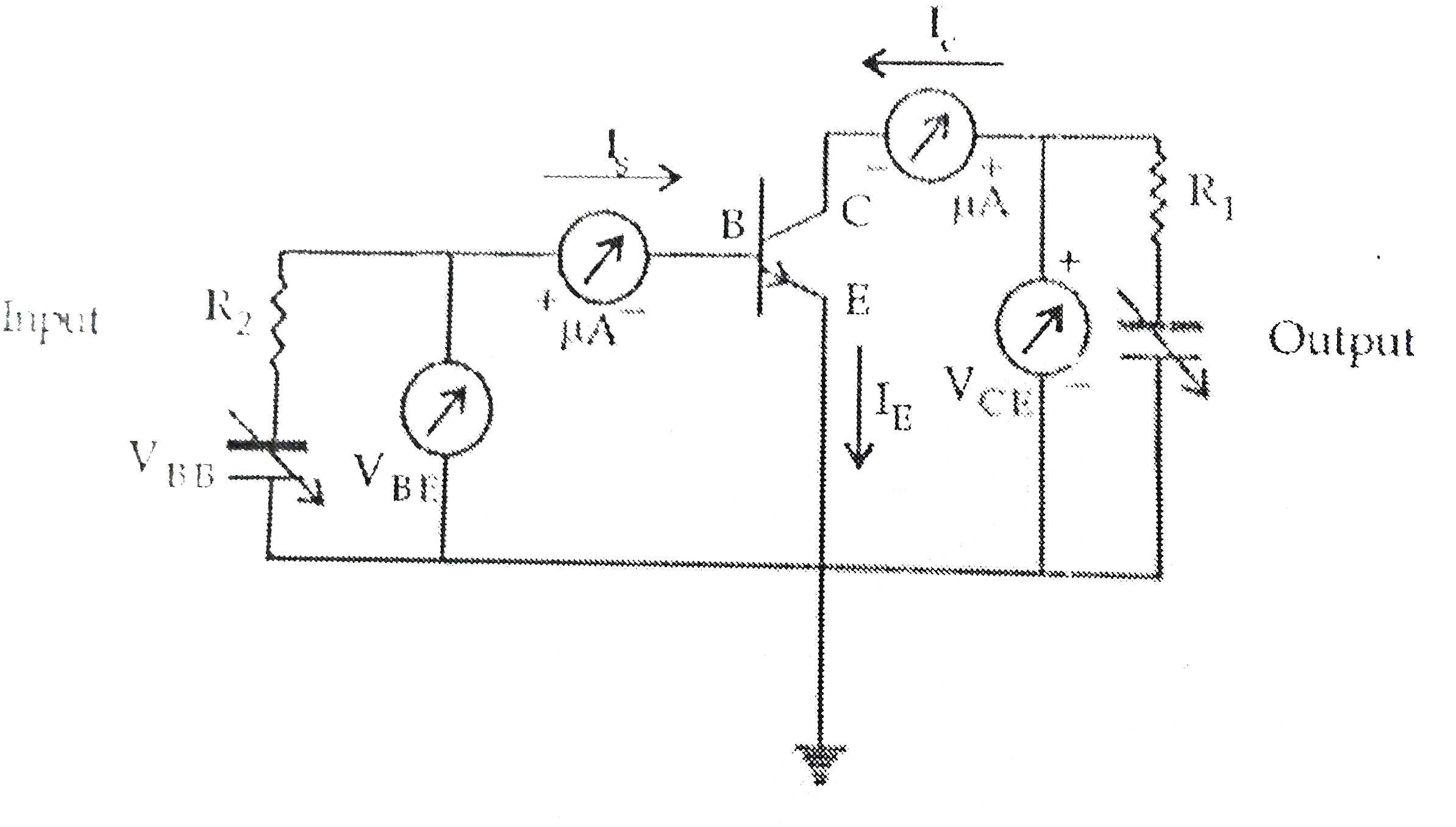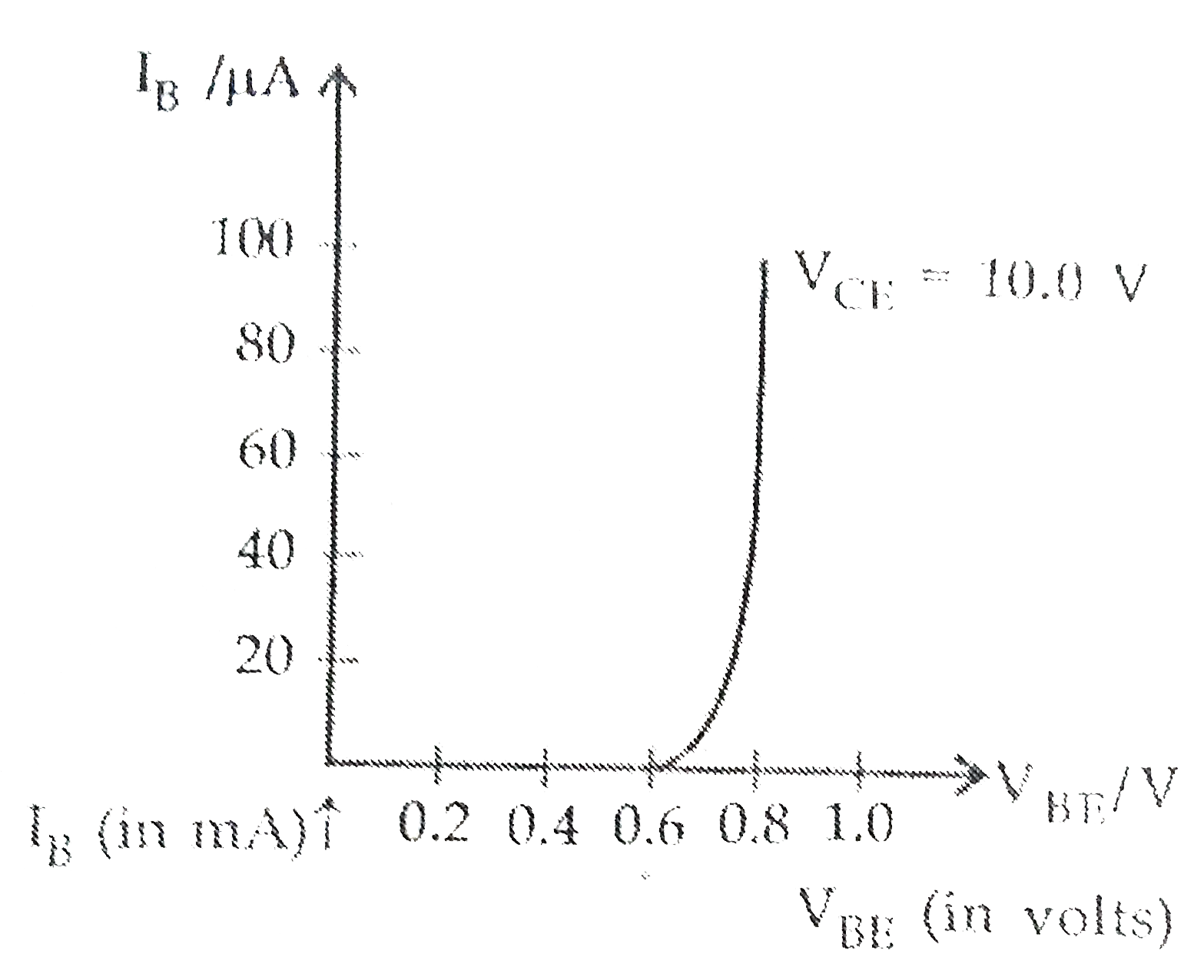# (a) Draw the circuit arrangement for studying the input and output characteristics of an n-p-n transistor in CE configuration . With the help of these

68 views
in Physics
closed
(a) Draw the circuit arrangement for studying the input and output characteristics of an n-p-n transistor in CE configuration . With the help of these characteristics define (i) input resistance , (ii) current amplification factor .
(b) Describe briefly with the help of a circuit diagram how an n-p-n transistor is used to produce self-sustained oscillations .

by (87.7k points)
selected

(a) Common emitter (CE) transistor characteristic : The transistor is most widely used in the CE configuration . When a transistor is used in CE configuration , the input is between the base and emitter and the output is between the collector and emitter .
The input and output characteristics of an n-p-n transistor in CE configuration can be studied by using the given circuit .(i) Input characteristics : The variation of the base current I_(B) with the base emitter voltage V_(BE) is called the input characteristics keeping V_(CE) fixed . A curve is plotted between the base current I_(B) against the base emitter voltage V_(CE) is kept fixed .
Since V_(CE) = V_(CB) + V_(BE) and for Silicon (Si) transistor V_(BE) is 0.6 to 0.7 V , V_(CE) must be larger than 0.7 V . The input characteristics of a transistor is shown in fig. (a) .
(ii) Output characteristics : The variations of the collector current I_(C) with the collector emitter voltage V_(CE) , keeping the base current I_(B) constant is called output characteristics .
The plot of I_(C) versus V_(CE) for different fixed values of I_(B) gives one output characteristic . The different output characteristics for different values of I_(B) is shown in ltbegt(iii) Input Resistance : This is defined as the ratio of the change in collector-emitter voltage (DeltaV_(CE)) to the resulting change in base current (Delta I_(B)) at constant collector-emitter voltage (V_(CE)).
therefore " " r_(i) = ((DeltaV_(BE))/(DeltaI_(B)))_(V_(CE))
(iv) Output Resistance : This is defined as the ratio of the change in base-emitter voltage (Delta_(VE)) to the change in collector current (DeltaI_(C)) at constant base current I_(B).
therefore " " r_(0) = ((DeltaV_(CE))/(DeltaI_(C)))_(I_(B))
(v) Current Amplification Factor (beta): This is defined as the ratio of the change in collector current (DeltaI_(C)) to change in base current (Delta I_(B)) at constant V_(CB).
beta_(ac) = ((DeltaI_(C))/(DeltaI_(B)))_(V_(CE))
This is also known as current gain .